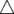Krishna
0

Step 1: Observe the given figures

Step 2: Find the corresponding angles of these congruent shapes.

NOTE: Corresponding parts are pairs of matching parts in congruent figures.

EXAMPLE:  \ \angle I=\angle P

\angle I=\angle P

\angle I=\angle P

Step 3 : Use these corresponding angles, in order, to write a congruence statement.

NOTE: A congruence statement says that two polygons are congruent.

To write a congruence statement, list the corresponding vertices in

the same order.

EXAMPLE :IJK ≅PQR

Step 4: Because these shapes have symmetry, there is more than one correct answer. You could also write:IJK ≅QPR